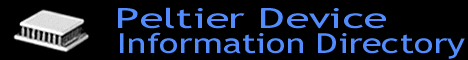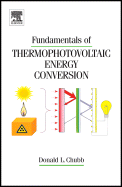# Peltier Device Information Directory

### (Thermoelectric Cooler/Heater/Generator Modules)Fundamentals of Thermophotovoltaic Energy Conversion

by Donald Chubb
Publisher: Elsevier
530 pages

From the publisher:

"This is a text book presenting the fundamentals of thermophotovoltaic(TPV) energy conversion suitable for an upper undergraduate or first year graduate course. In addition it can serve as a reference or design aid for engineers developing TPV systems. Mathematica design programs for interference filters and a planar TPV system are included on a CD-Rom disk. Each chapter includes a summary and concludes with a set of problems. The first chapter presents the electromagnetic theory and radiation transfer theory necessary to calculate the optical properties of the components in a TPV optical cavity. Using a simplified model, Chapter 2 develops expressions for the maximum efficiency and power density for an ideal TPV system. The next three chapters consider the three major components in a TPV system; the emitter, filter and photovoltaic(PV) array. Chapter 3 applies the electromagnetic theory and radiation transfer theory presented in Chapter 1 in the calculation of spectral emittance. From the spectral emittance the emitter efficiency is calculated. Chapter 4 discusses interference, plasma and resonant array filters plus an interference filter with an imbedded metallic layer, a combined interference-plasma filter and spectral control using a back surface reflector(BSR) on the PV array. The theory necessary to calculate the optical properties of these filters is presented. Chapter 5 presents the fundamentals of semiconductor PV cells. Using transport equations calculation of the current-voltage relation for a PV cell is carried out. Quantum efficiency, spectral response and the electrical equivalent circuit for a PV cell are introduced so that the PV cell efficiency and power output can be calculated. The final three chapters of the book consider the combination of the emitter, filter and PV array that make up the optical cavity of a TPV system. Chapter 6 applies radiation transfer theory to calculate the cavity efficiency of planar and cylindrical optical cavities. Also introduced in Chapter 6 are the overall TPV efficiency, thermal efficiency and PV efficiency. Leakage of radiation out of the optical cavity results in a significant loss in TPV efficiency. Chapter 7 considers that topic. The final chapter presents a model for a planar TPV system. Six appendices present background information necessary to carry out theoretical developments in the text. Two of the appendices include Mathematica programs for the spectral optical properties of multi-layer interference filters and a planar TPV system. These programs are contained on a CD-Rom disk included with the book."